# Active Filters

• #### Question 1

 Don’t just sit there! Build something!!

Learning to mathematically analyze circuits requires much study and practice. Typically, students practice by working through lots of sample problems and checking their answers against those provided by the textbook or the instructor. While this is good, there is a much better way.

You will learn much more by actually building and analyzing real circuits, letting your test equipment provide the “answers” instead of a book or another person. For successful circuit-building exercises, follow these steps:

1. Carefully measure and record all component values prior to circuit construction.
2. Draw the schematic diagram for the circuit to be analyzed.
3. Carefully build this circuit on a breadboard or other convenient medium.
4. Check the accuracy of the circuit’s construction, following each wire to each connection point, and verifying these elements one-by-one on the diagram.
5. Mathematically analyze the circuit, solving for all voltage and current values.
6. Carefully measure all voltages and currents, to verify the accuracy of your analysis.
7. If there are any substantial errors (greater than a few percent), carefully check your circuit’s construction against the diagram, then carefully re-calculate the values and re-measure.

Avoid using the model 741 op-amp, unless you want to challenge your circuit design skills. There are more versatile op-amp models commonly available for the beginner. I recommend the LM324 for DC and low-frequency AC circuits, and the TL082 for AC projects involving audio or higher frequencies.

As usual, avoid very high and very low resistor values, to avoid measurement errors caused by meter “loading”. I recommend resistor values between 1 kΩ and 100 kΩ.

One way you can save time and reduce the possibility of error is to begin with a very simple circuit and incrementally add components to increase its complexity after each analysis, rather than building a whole new circuit for each practice problem. Another time-saving technique is to re-use the same components in a variety of different circuit configurations. This way, you won’t have to measure any component’s value more than once.

• #### Question 2

In very simple, qualitative terms, rate the impedance of capacitors and inductors as “seen” by low-frequency and high-frequency signals alike:

Capacitor as it “appears” to a low frequency signal: (high or low) impedance?
Capacitor as it “appears” to a high frequency signal: (high or low) impedance?
Inductor as it “appears” to a low frequency signal: (high or low) impedance?
Inductor as it “appears” to a high frequency signal: (high or low) impedance?
• #### Question 3

Draw the Bode plot for an ideal high-pass filter circuit:Be sure to note the “cutoff frequency” on your plot.

• #### Question 4

Draw the Bode plot for an ideal low-pass filter circuit: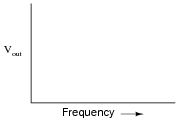Be sure to note the “cutoff frequency” on your plot.

• #### Question 5

Identify what type of filter this circuit is, and calculate its cutoff frequency given a resistor value of 1 kΩ and a capacitor value of 0.22 μF: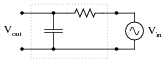Calculate the impedance of both the resistor and the capacitor at this frequency. What do you notice about these two impedance values?

• #### Question 6

Real filters never exhibit perfect “square-edge” Bode plot responses. A typical low-pass filter circuit, for example, might have a frequency response that looks like this: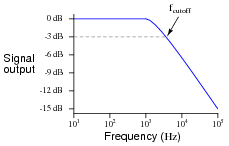What does the term rolloff refer to, in the context of filter circuits and Bode plots? Why would this parameter be important to a technician or engineer?

• #### Question 7

Identify what factor(s) determine the cutoff frequency of this passive filter circuit: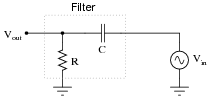Give an exact equation predicting this filter circuit’s cutoff frequency, and also identify what type of filter it is.

• #### Question 8

In this passive filter circuit, how will the filter’s cutoff frequency be affected by changes in the load resistance? Be as specific as you can in your answer.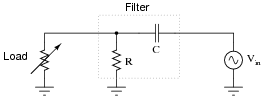• #### Question 9

In this active filter circuit, how will the filter’s cutoff frequency be affected by changes in the load resistance? Be as specific as you can in your answer.• #### Question 10

In this filter circuit, how will the filter’s cutoff frequency be affected by changes in the potentiometer position? Be as specific as you can in your answer.• #### Question 11

Determine the type (LP, HP, BP, BS) and cutoff frequency of this active filter circuit:• #### Question 12

Determine the type (LP, HP, BP, BS) and cutoff frequency of this active filter circuit: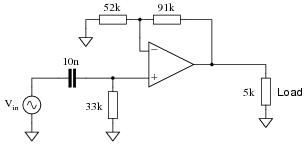• #### Question 13

Compare the voltage gains of these two opamp circuits: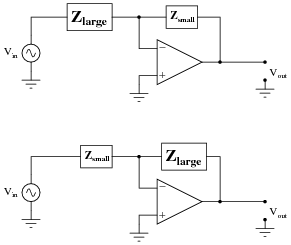Which one has the greater AV, and why?

• #### Question 14

Describe what will happen to the impedance of both the capacitor and the resistor as the input signal frequency increases:Also, describe what result the change in impedances will have on the op-amp circuit’s voltage gain. If the input signal amplitude remains constant as frequency increases, what will happen to the amplitude of the output voltage? What type of filtering function does this behavior represent?

• #### Question 15

Describe what will happen to the impedance of both the capacitor and the resistor as the input signal frequency increases:Also, describe what result the change in impedances will have on the op-amp circuit’s voltage gain. If the input signal amplitude remains constant as frequency increases, what will happen to the amplitude of the output voltage? What type of filtering function does this behavior represent?

• #### Question 16

Approximate the voltage gains of this active filter circuit at f = 0 and f = ∞ (assume ideal op-amp behavior):Approximate the voltage gains of this other “active filter” circuit at f = 0 and f = ∞ (assume ideal op-amp behavior):What type of filtering function (low pass, high pass, band pass, band stop) is provided by both these filter circuits? Comparing these two circuit designs, which one do you think is more practical? Explain your answer.

• #### Question 17

Approximate the voltage gains of this active filter circuit at f = 0 and f = ∞ (assume ideal op-amp behavior):Approximate the voltage gains of this other “active filter” circuit at f = 0 and f = ∞ (assume ideal op-amp behavior):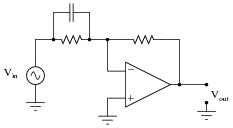What type of filtering function (low pass, high pass, band pass, band stop) is provided by both these filter circuits? Comparing these two circuit designs, which one do you think is more practical? Explain your answer.

• #### Question 18

Identify the function of this active filter: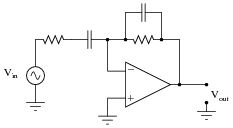It is low pass, high pass, band pass, or band stop? Explain your answer.

• #### Question 19

Identify the function of this active filter: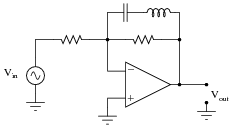It is low pass, high pass, band pass, or band stop? Explain your answer.

• #### Question 20

A very popular active filter topology is called the Sallen-Key. Two examples of Sallen-Key active filter circuits are shown here: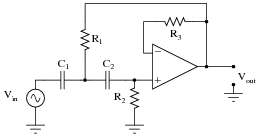Determine which of these Sallen-Key filters is low pass, and which is high pass. Explain your answers.

• #### Question 21

In active and passive filter design literature, you often come across filter circuits classified as one of three different names:

Chebyshev
Butterworth
Bessel

Describe what each of these names means. What, exactly, distinguishes a “Chebyshev” filter circuit from a “Butterworth” filter circuit?

• #### Question 22

Choose appropriate values for this Sallen-Key high-pass filter circuit to give it a cutoff frequency of 7 kHz with a “Butterworth” response:f−3dB = √2 2 πR C

A good guideline to follow is to make sure no component impedance (ZR or ZC) at the cutoff frequency is less than 1 kΩ or greater than 100 kΩ.

• #### Question 23

Choose appropriate values for this Sallen-Key low-pass filter circuit to give it a cutoff frequency of 4.2 kHz with a “Butterworth” response: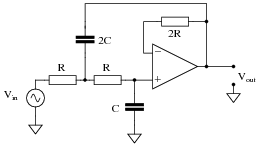f−3dB = 1 2 √2 πR C

A good guideline to follow is to make sure no component impedance (ZR or ZC) at the cutoff frequency is less than 1 kΩ or greater than 100 kΩ.

• #### Question 24

A popular passive filtering network called the twin-tee is often coupled with an operational amplifier to produce an active filter circuit. Two examples are shown here: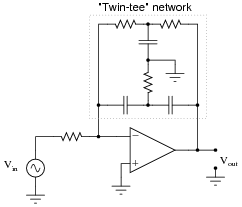Identify which of these circuits is band-pass, and which is band-stop. Also, identify the type of response typically provided by the twin-tee network alone, and how that response is exploited to make two different types of active filter responses.

• #### Question 25

Singers who wish to practice singing to popular music find that the following vocal eliminator circuit is useful: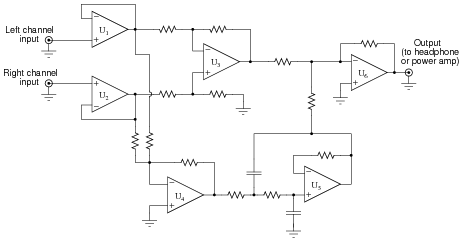The circuit works on the principle that vocal tracks are usually recorded through a single microphone at the recording studio, and thus are represented equally on each channel of a stereo sound system. This circuit effectively eliminates the vocal track from the song, leaving only the music to be heard through the headphone or speaker.

Operational amplifiers U1 and U2 provide input buffering so that the other opamp circuits do not excessively load the left and right channel input signals. Opamp U3 performs the subtraction function necessary to eliminate the vocal track.

You might think that these three opamps would be sufficient to make a vocal eliminator circuit, but there is one more necessary feature. Not only is the vocal track common to both left and right channels, but so is most of the bass (low-frequency) tones. Thus, the first three opamps (U1, U2, and U3) eliminate both vocal and bass signals from getting to the output, which is not what we want.

Explain how the other three opamps (U4, U5, and U6) work to restore bass tones to the output so they are not lost along with the vocal track.

• #### Question 26

Predict how the operation of this active filter circuit will be affected as a result of the following faults. Consider each fault independently (i.e. one at a time, no multiple faults):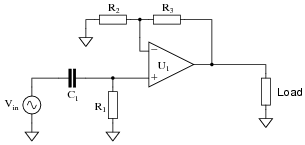Resistor R1 fails open:
Capacitor C1 fails open:
Solder bridge (short) across resistor R1:
Solder bridge (short) across capacitor C1:
Resistor R2 fails open:
Resistor R3 fails open:

For each of these conditions, explain why the resulting effects will occur.

• #### Question 27

Predict how the operation of this active filter circuit will be affected as a result of the following faults. Consider each fault independently (i.e. one at a time, no multiple faults):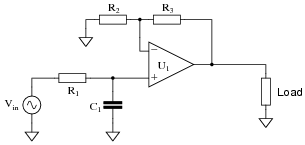Resistor R1 fails open:
Capacitor C1 fails open:
Solder bridge (short) across resistor R1:
Solder bridge (short) across capacitor C1:
Resistor R2 fails open:
Resistor R3 fails open:

For each of these conditions, explain why the resulting effects will occur.

• #### Question 28

Predict how the operation of this active differentiator circuit will be affected as a result of the following faults. Consider each fault independently (i.e. one at a time, no multiple faults):Resistor R1 fails open:
Capacitor C1 fails open:
Solder bridge (short) across resistor R1:
Solder bridge (short) across capacitor C1:

For each of these conditions, explain why the resulting effects will occur.

• #### Question 29

Predict how the operation of this active filter circuit will be affected as a result of the following faults. Consider each fault independently (i.e. one at a time, no multiple faults):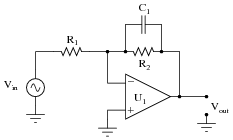Resistor R1 fails open:
Resistor R2 fails open:
Capacitor C1 fails open:
Solder bridge (short) across resistor R1:
Solder bridge (short) across resistor R2:
Solder bridge (short) across capacitor C1:

For each of these conditions, explain why the resulting effects will occur.

• #### Question 30

Predict how the operation of this active filter circuit will be affected as a result of the following faults. Consider each fault independently (i.e. one at a time, no multiple faults):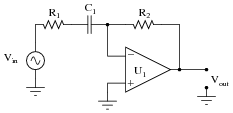Resistor R1 fails open:
Resistor R2 fails open:
Capacitor C1 fails open:
Solder bridge (short) across resistor R1:
Solder bridge (short) across resistor R2:
Solder bridge (short) across capacitor C1:

For each of these conditions, explain why the resulting effects will occur.

• #### Question 31

This vocal eliminator circuit used to work just fine, but then one day it seemed to lose a lot of its bass. It still did its job of eliminating the vocal track, but instead of hearing the full range of musical tones it only reproduced the high frequencies, while the low frequency tones were lost: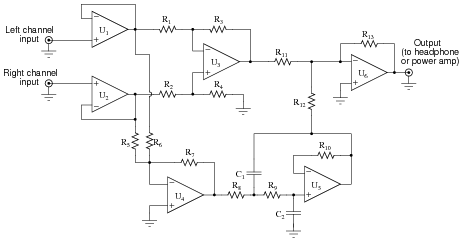Identify the following fault possibilities:

One resistor failure (either open or shorted) that could cause this to happen:

One capacitor failure (either open or shorted) that could cause this to happen:

One opamp failure that could cause this to happen:

For each of these proposed faults, explain why the bass tones would be lost.

• #### Question 32

This vocal eliminator circuit used to work just fine, but then one day it stopped eliminating the vocal track. The tone of the music sounded a bit heavy on the bass, and the vocal track was there when it shouldn’t have been there:Identify the following fault possibilities:

One resistor failure (either open or shorted) that could cause this to happen:

One opamp failure that could cause this to happen:

For each of these proposed faults, explain why the bass tones would be lost.